How to Calculate and Solve for the Volume of a Spherical Segment | Nickzom Calculator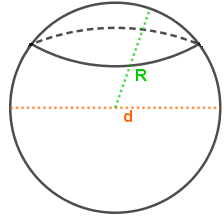The image above is a spherical segment.

To compute the volume of a spherical segment requires three essential parameters which are the radius of the spherical segment base (r1), radius of the spherical segment (r2) and height (h).

The formula for calculating the volume of the spherical segment:

V = πh(3r1² + 3r2² + h²)6

Where;
V = Volume of the spherical cap
r1 = Radius of the spherical segment base
r2 = Radius of the spherical segment base
h = Height of the spherical segment

Let’s solve an example;
Find the volume of a spherical segment when the radius of the spherical segment base (r1) is 7 cm, radius of the spherical segment base (r2) is 9 cm and a height of 20 cm.

This implies that;
r1 = Radius of the spherical segment base = 7 cm
r2 = Radius of the spherical segment base = 9 cm
h = Height of the spherical segment = 20 cm

V = πh(3r1² + 3r2² + h²)6
V = π x 20(3 x 7² + 3 x 9² + 20²)6
V = π x 20(3 x 49 + 3 x 81 + 400)6
V = π x 20(147 + 243 + 400)6
V = π x 20(790)6
V = π x 158006
V = 49643.66
V = 8273.9

Therefore, the volume of the spherical segment is 8273.9 cm3.

How to Calculate the Radius, Height and Curved Surface Area of a Spherical Cap | The Calculator Encyclopedia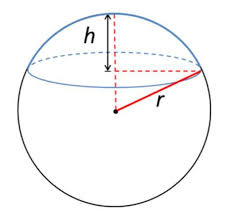The image above is a spherical cap.

To compute the curved surface area of a spherical cap requires two essential parameters which are the radius of the base of the cap (a) and the height (h).

The formula for calculating the curved surface area of the spherical cap:

A = π(a² + h²)

Where;
A = Curved surface area of the spherical cap
a = Radius of the base of the cap
h = Height of the spherical cap

Let’s solve an example;
Find the curved surface area of a spherical cap with radius of the base 7 cm and the height of 13 cm.

This implies that;
a = Radius of the base of the cap = 7 cm
h = Height of the spherical cap = 13 cm

A = π(a² + h²)
A =  π(7² + 13²)
A =  π(49 + 169)
A =  π(218)
A = 684.867

Therefore, the curved surface area of the spherical cap is 684.867 cm².

Calculating the Radius of the base of a Spherical Cap using the Curved Surface Area of the Spherical Cap and the Height.

a = √A – πh2 / π

Where;
A = Curved surface area of the spherical cap
a = Radius of the base of the cap
h = Height of the spherical cap

Let’s solve an example;
Find the radius of the base of a spherical cap when the curved surface area of the spherical cap is 300 cm2 and a height of 7 cm.

This implies that;
A = Curved surface area of the spherical cap = 300 cm2
h = Height of the spherical cap = 7 cm

a = √A – πh2 / π
a = √300 – 3.142 x 72 / π
a = √300 – 3.142 x 49 / π
a = √300 – 153.958 / π
a = √146.042 / π
a = √46.48
a = 6.82

Therefore, the radius of the base of the cap is 6.82 cm.

How to Calculate and Solve for the Volume of a Spherical Cap | Nickzom Calculator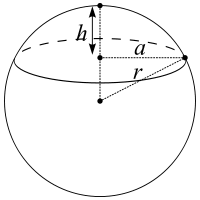The image above is a spherical cap.

To compute the volume of a spherical cap requires two essential parameters which are the radius of the base of the cap (a) and the height (h).

The formula for calculating the volume of the spherical cap:

V = πh(3a² + h²) ⁄ 6

Where;
V = Volume of the spherical cap
a = Radius of the base of the cap
h = Height of the spherical cap

Lets solve an example;
Find the volume of a spherical cap when the radius of the base is 12 cm and the height is 22 cm.

This implies that;
a = Radius of the base of the cap = 12 cm
h = Height of the spherical cap = 22 cm

V = πh(3a² + h²) ⁄ 6
V = 3.142 (22)(3(12)² + 22²) ⁄ 6
V = 3.142 (22)(3(144) + 484) ⁄ 6
V = 3.142 (22)(432 + 484) ⁄ 6
V = 3.142 (22)(916) ⁄ 6
V = 3.142 (20152)6
V = 63309.37 ⁄ 6
V = 10551.56

Therefore, the volume of the spherical cap is 10551.56 cm2.

How to Calculate and Solve for the Total Surface Area of a Conical Frustum | The Calculator Encyclopedia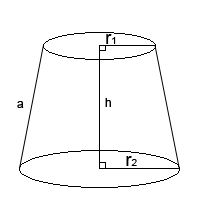The image above is a conical frustum.

To compute the total surface area of a conical frustum requires three essential parameters which are the radius of the lower base (R), radius of the upper base (r) and the height (h).

The formula for calculating the total surface area of a conical frustum;

A = π[R² + r² + (R + r)√((R – r)² + h²)]

Where;
A = Total surface area of the conical frustum
R = Radius of the lower base
r = Radius of the upper base
h = Height of the conical frustum

Let’s solve an example;
Find the total surface area of the conical frustum when the radius of the upper base is 11 cm, radius of the lower base is 17 cm and the height is 30 cm.

This implies that;
r = Radius of the upper base = 11 cm
R = Radius of the lower base = 17 cm
h = Height of the conical frustum = 30 cm

A = π[R² + r² + (R + r)√((R – r)² + h²)]
A = π[17² + 11² + (17 + 11)√((17 – 11)² + 30²)]
A = π[289 + 121 + (28)√((6)² + 900)]
A = π[289 + 121 + (28)√(36+ 900)]
A = π[289 + 121 + (28)√(936)]
A = π[289 + 121 + (28)(30.59)]
A = π[289 + 121 + 856.63]
A = π[1266.63]
A = 3979.25

Therefore, the total surface area of the conical frustum is 3979.25 cm².

How to Calculate and Solve for the Lateral Surface Area of a Conical Frustum | Nickzom Calculator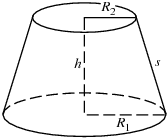The image above is a conical frustum.

To compute the lateral surface area of a conical frustum requires three essential parameters which are the radius of the lower base (R), radius of the upper base (r) and the height (h).

The formula for calculating the lateral surface area of a conical frustum:

A = π(R + r)√((R – r)² + h²)

Where;
A = Area of the conical frustum
R = Radius of the lower base
r = Radius of the upper base
h = Height of the conical frustum

Let’s solve an example;
Given that the height of a conical frustum is 28 cm with a radius of lower base of 22 cm and a radius of upper base of 19 cm. Find the lateral surface area of the conical frustum?

This implies that;
h = Height of the conical frustum = 28 cm
R = Radius of the lower base = 22 cm
r = Radius of the upper base = 19 cm

A = π(R + r)√((R – r)² + h²)
A = 3.142(22 + 19)√((22 – 19)² + 28²)
A = 3.142(41)√((3)² + 28²)
A = 3.142 (41)√(9 + 784)
A = 3.142 (41)√(793)
A = 3.142 (41)(28.16)
A = 3.142 x 1154.56
A = 3627.63

Therefore, the lateral surface area of the conical frustum is 3627.63 cm².

How to Calculate and Solve for the Width, Height, Length and Area of a Rectangular Prism | The Calculator Encyclopedia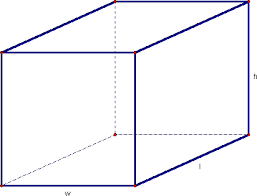The image above is a rectangular prism.

To compute the area of a rectangular prism requires three essential parameters which are the length, width and height of the rectangular prism.

A = 2wl + 2hl + 2hw

Where;
A = Area of the rectangular prism
l = Length of the rectangular prism
w = Width of the rectangular prism
h = Height of the rectangular prism

Let’s solve an example;
Find the area of a rectangular prism with a width of 10 cm, a height of 17 cm and a length of 14 cm.

This implies that;
l = Length of the rectangular prism = 14 cm
w = Width of the rectangular prism = 10 cm
h = Height of the rectangular prism = 17 cm

A = 2wl + 2hl + 2hw
A = 2 x 10 x 14 + 2 x 17 x 14 + 2 x 17 x 10
A = 280 + 476 + 340
A = 1096

Therefore, the area of the rectangular prism is 1096 cm2.

Calculating the Length of a Rectangular Prism using the Area, Width and Height of the Rectangular Prism.

l = A – 2hw / 2 (w + h)

Where;
l = Length of the rectangular prism
A = Area of the rectangular prism
w = Width of the rectangular prism
h = Height of the rectangular prism

Let’s solve an example;
Find the length of a rectangular prism with a width of 9 cm, a height of 12 cm and a Area of 250 cm2.

This implies that;
w = Width of the rectangular prism = 9 cm
h = Height of the rectangular prism = 12 cm
A = Area of the rectangular prism = 250 cm2

l = A – 2hw / 2 (w + h)
l = 250 – 2 x 12 x 9 / 2 (9 + 12)
l = 250 – 216 / 2 (21)
l = 34 / 42
l = 0.81

Therefore, the length of the rectangular prism is 0.81 cm.

How to Calculate and Solve for the Width, Height, Length and Volume of a Rectangular Prism | Nickzom CalculatorThe image above is a rectangular prism.

To compute the volume of a rectangular prism requires three essential parameters which are the length, width and height of the rectangular prism.

V = lwh

Where;
V = Volume of the rectangular prism
l = Length of the rectangular prism
w = Width of the rectangular prism
h = Height of the rectangular prism

Let’s solve an example;
Find the volume of a rectangular prism with a length of 18 cm, a width of 12 cm and a height of 21 cm.

This implies that;
l = Length of the rectangular prism = 18 cm
w = Width of the rectangular prism = 12 cm
h = Height of the rectangular prism = 21 cm

V = lwh
V = 18 x 12 x 21
V = 4536

Therefore, the volume of the rectangular prism is 4536 cm3.

Calculating the Length of a Rectangular Prism using the Volume, Width and Height of the Rectangular Prism.

l = V / wh

Where;
l = Length of the rectangular prism
V = Volume of the rectangular prism
w = Width of the rectangular prism
h = Height of the rectangular prism

Let’s solve an example;
Find the length of a rectangular prism with a width of 19 cm, a height of 24 cm and a Volume of 250 cm3.

This implies that;
w = Width of the rectangular prism = 19 cm
h = Height of the rectangular prism = 24 cm
V = Volume of the rectangular prism = 250 cm3

l = V / wh
l = 250 / 19 x 24
l = 250 / 456
l = 0.55

Therefore, the length of the rectangular prism is 0.55 cm.

How to Calculate and Solve for the Length of a Side and Perimeter of a Cube | The Calculator Encyclopedia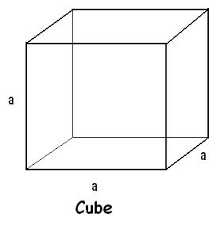The image above is a cube.

To compute the perimeter of a cube, one essential parameter is needed and this parameter is length of one of the sides (a).

The formula for calculating the perimeter of a cube:

P = 12a

Where;
P = Perimeter of the cube
a = Length of one of the sides of the cube

Let’s solve an example;
Find the perimeter of a cube when the length of one of the sides is 14 cm.

This implies that;
a = Length of one of the sides = 14 cm

P = 12a
P = 12 x 14
P = 168

Therefore, the perimeter of the cube is 168 cm.

Calculating the Length of one of the sides of a Cube using the Perimeter of the Cube.

a = P / 12

Where;
a = Length of one of the sides of the cube
P = Perimeter of the cube

Let’s solve an example;
Find the length of one of the sides of a cube when the perimeter of the cube is 200 cm.

This implies that;
P = Perimeter of the cube = 200 cm

a = P / 12
a = 200 / 12
a = 16.67

Therefore, the length of one of the sides is 16.67 cm.

How to Calculate and Solve for the Perimeter of an Ellipse | Nickzom Calculator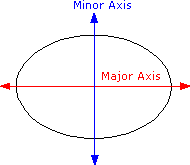The image above is an ellipse.

To compute the perimeter of an ellipse, two essential parameters are needed and this parameters are axis (a) and axis (b).

The formula for calculating the perimeter of an ellipse:

P = π [3(a + b) – √((3a + b)(a + 3b))]

Where;

P = Perimeter of the ellipse
a = Axis of the ellipse
b = Axis of the ellipse

Let’s solve an example;
Given that the axis of the ellipse (a) is 19 cm and axis of the ellipse (b) is 31 cm. Find the perimeter of the ellipse?

This implies that;
a = Axis of the ellipse = 19 cm
b = Axis of the ellipse = 31 cm

P = π [3(a + b) – √((3a + b)(a + 3b))]
P = 3.142 [3(19 + 31) – √((3 x 19 + 31)(19 + 3 x 31))]
P = 3.142 [3(50) – √((57+ 31)(19 + 93))]
P = 3.142 [150 – √((88)(112))]
P = 3.142 [150 – √(9856)]
P = 3.142 [150 – 99.28]
P = 3.142 [50.72]
P = 3.142 x 50.72
P = 159.26

Therefore, the perimeter of the ellipse is 159.26 cm.

How to Calculate and Solve for the Axis and Area of an Ellipse | Nickzom Calculator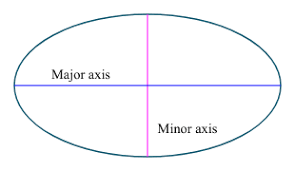The image above is an ellipse.

To compute the area of an ellipse, two essential parameters are needed and this parameters are axis (a) and axis (b).

A = πab

Where;
A = Area of the ellipse
a = Axis of the ellipse
b = Axis of the ellipse

Let’s solve an example;
Find the area of an ellipse when it has an axis (a) of 10 cm and an axis (b) of 17 cm.

This implies that;
a = Axis of the ellipse = 10 cm
b = Axis of the ellipse = 17 cm

A = πab
A = 3.142 x 10 x 17
A = 534.14

Therefore, the area of the ellipse is 534.14 cm2.

Calculating the Axis (a) of an ellipse using Area of the ellipse and Axis (b) of the ellipse.

a = A / πb

Where;
a = Axis of the ellipse
A = Area of the ellipse
b = Axis of the ellipse

Let’s solve an example;
Find the axis (a) of an ellipse when the area of the ellipse is 210 cm2 with an axis (b) of 19 cm.

This implies that;
A = Area of the ellipse = 210 cm2
b = Axis of the ellipse = 19 cm

a = A / πb
a = 210 / 3.142 x 19
a = 210 / 59.70
a = 3.52

Therefore, the axis (a) of an ellipse is 3.52 cm.